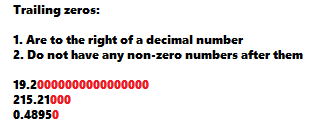# Significant Figures

## What are Significant Figuress

Significant figures (also called “sig figs” or significant digits) is a count of a number’s important or interesting digits.

For example:

• 0.0035 = 2 significant digits. The zeros in front (called “leading zeros” don’t count!),
• 563 = 3 significant digits,
• 7839 = 4 significant digits,
• 2,040.3 = 5 significant digits,

## Significant Figures & Leading and Trailing zeros

Leading zeros, as noted above, don’t count. Only discount the zeros to the left of the first non-zero digit. For example, both of these numbers have just one significant digit (which is “2”):
00000000000002
and
002.Trailing zeros aren’t as easy to ignore. Sometimes they are counted and sometimes they aren’t counted. In general, if you know your figure is accurately measured, then count the zeros. If it’s rounded, then don’t count them. For example:

• A truck pulls into a weight station and weighs exactly 9,000 pounds. This is 4 significant figures, because you know it’s an accurate measure.
• You weigh a substance and the weight is 323.0 ounces. This is 4 significant digits. The zero after the decimal point is measured accurately.
• A friend reports she weighs 200 pounds. This is only one significant figure. Your friend probably rounded up or down.

## Rounding Numbers for Significant Figures

When you round a number, you shorten it to a certain number of significant figures. A general rule of thumb is to round to the number of digits in the largest (as in, the largest number of digits, not the highest on value the number line) number.

Example: You have \$50 to divide between 6 nieces and nephews. 50/6 = 8.33333333333, which isn’t very practical. An alternative is to round to two significant digits: 8.4. Why two? Because the largest number you’re dealing with is 50, and that number has two sig figs.

Example of how to round 9.862 to 3 sig figs:

Step 1: Draw a line before the 3rd digit: 9.8|62.

Step 2: Round the first digit after the line up or down. If the first digit after the line is 0 to 4, round down and if it’s 5 to 9, round up. The first digit here is is a 6, so round up and remove any numbers after that place: 9.87.

## Multiplying and Dividing

Q. How do I round when multiplying and dividing?
A. Round the largest value to that value’s number of significant figures. For example:

• 2587 / 352 = 7.349 rounded to four places,
• 23/7 = 3.3 rounded to two places.

## Roots and Powers

Do not round roots or powers. For example:
√324.34 = 5 places
2.32 = 2 places

## General Rounding Rule for Reports

The advice is a little vague for reporting results, with differing opinions, depending on who you ask. In general, round to one more decimal place than the least precise number. For example, if you have 2, 4.3, 11.2, 3, 4.5, then round to one decimal place (the whole numbers are the least precise with zero places).

CITE THIS AS:
Stephanie Glen. "Significant Figures" From StatisticsHowTo.com: Elementary Statistics for the rest of us! https://www.statisticshowto.com/significant-figures/